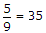# Online Electronics and Communication Engineering Test - Microprocessors TestLoading Test...

Instruction:

• This is a FREE online test. DO NOT pay money to anyone to attend this test.
• Total number of questions : 20.
• Time alloted : 30 minutes.
• Each question carry 1 mark, no negative marks.
• DO NOT refresh the page.
• All the best :-).

1.

Which of the following is not a key word in C?

A.
 popB.
 forC.
 ifD.
 unionExplanation:

pop is not a keyword in C.

2.

A computer program is used to read M and print the sum 2 + 4 + 6 + 8 +.........+ 2M. If M = 9 , the print out will show the number

A.
 9B.
 18C.
 90D.
 100Explanation:

SUM = 2 + 4 + 6 + 8 + 10 + 12 + 14 + 16 + 18 = 90.

3.

Consider the following statements about an array in Pascal

1. An array has a fixed predetermined number of components.
2. Any component of an array can be accessed using an index.
3. An array variable name refers to a group of quantities by a single name.
4. When array variables are used an appropriate declaration is required.
Which of the above are correct?

A.
 AllB.
 1, 2, 3 onlyC.
 2, 3, 4 onlyD.
 1, 2 and 4 onlyExplanation:

All are true.

4.

If F = 95.0 what will be the result of following FORTRAN 77 program? `REAL F , CREAD * , FC = (5. / 9.) * (F - 32.)`

A.
 35B.
 35C.
 25D.
 25Explanation:

(95 - 32)and decimal must be added.

5.

Consider the following expressions in Java
`int a, b, c, a = 40;b = a++ ;c = ++a ;`
Now the values of a, b, c are

A.
 42, 40, 42 respectivelyB.
 42, 42, 42 respectivelyC.
 40, 42, 42 respectivelyD.
 40, 40, 42 respectivelyExplanation:

The steps are : a is given the value 40, b is given the value of a before it is incremented (i.e., 40) and a is incremented to 41, a is incremented to 42 and c is given this value. Thus the result is 42, 40, 42.

6.

The number of primitive string operations in 8086 is

A.
 3B.
 4C.
 5D.
 67.

The standard FORTRAN exponential form of decimal number - 0.000000023 is

A.
 2.3 E - 8B.
 -2.3 E - 8C.
 2.3 E - 7D.
 -2.3 E - 78.

Which of the following methods does not cause any reduction in instruction length?

A.
 Using program counterB.
 Common source and destination addressC.
 Implicit source and destination addressD.
 Machine language programming9.

Assertion (A): In C arithmetic operations are pemiissible on ints, floats and chars.

Reason (R): In C every arithmetic operator must be written explicitly.

A.
 Both A and R are correct and R is correct explanation of AB.
 Both A and R are correct but R is not correct explanation of AC.
 A is correct R is wrongD.
 A is wrong R is correct10.

Assertion (A): In microprocessor 8085 instruction LXIB, 90 FF H means register B and C are loaded with upper and lower bytes to get B = 90 H and C = FFH.

Reason (R): In 8085 the stack pointer indicates which memory location is to accessed.

A.
 Both A and R are correct and R is correct explanation of AB.
 Both A and R are correct but R is not correct explanation of AC.
 A is correct R is wrongD.
 A is wrong R is correct11.

The initial contents of `ACC and CY` in 8085 are

1. `CY = 1`
2. `ACC = 10000001`
After instruction `RAL` is executed once the contents of `CY and ACC` respectively will be

A.
 1 and 00000011B.
 0 and 00000011C.
 1 and 00000001D.
 0 and 0000000112.

The word size accessible at a time from the memory subsystem depends on

A.
 width of data busB.
 width of address busC.
 width of control busD.
 both (b) and (c)13.

8085 data bus has 8 lines and is bidirectional.

A.
 TrueB.
 False14.

A PC has typically

A.
 5 to 10 KB of main memoryB.
 10 to 100 KB of main memoryC.
 100 to 256 KB of main memoryD.
 256 KB to 1 MB of main memory15.

RAM is a volatile memory device.

A.
 TrueB.
 False16.

1. Multi-processing operation permits highly efficient parallel processing at several levels.
2. Multi processing involves use of several processing units jointly on a common program and severally on different programs.
3. In multi-processing the assignment of facilities to tasks, loading of programs and monitoring of I/O activity is taken care of by operating system.
Which of the above are correct?

A.
 AllB.
 1 and 2 onlyC.
 1 and 3 onlyD.
 2 and 3 only17.

Which memory has read operation, byte erase, byte write and chip erase?

A.
 RAMB.
 UVEPROMC.
 EEPROMD.
 both (b) and (c)18.

Assertion (A): Structures are useful in DBMS.

Reason (R): Structures are very large numbers of applications besides DBMS.

A.
 Both A and R are correct and R is correct explanation of AB.
 Both A and R are correct but R is not correct explanation of AC.
 A is correct R is wrongD.
 A is wrong R is correct19.

The use of microprocessor control in automobiles results in

A.
 improved fuel economyB.
 improved car controlC.
 advanced information system for driverD.
 all of the above20.

In a typical microcomputer data transfer takes place between

A.
 μP and memoryB.
 μP and I/O devicesC.
 memory and I/O devicesD.
 all of the above